Скачать презентацию Multiple Discriminant Analysis and Logistic Regression Multiple

f83e1838e4a9e9ffeec8e729e5b31820.ppt

• Количество слайдов: 61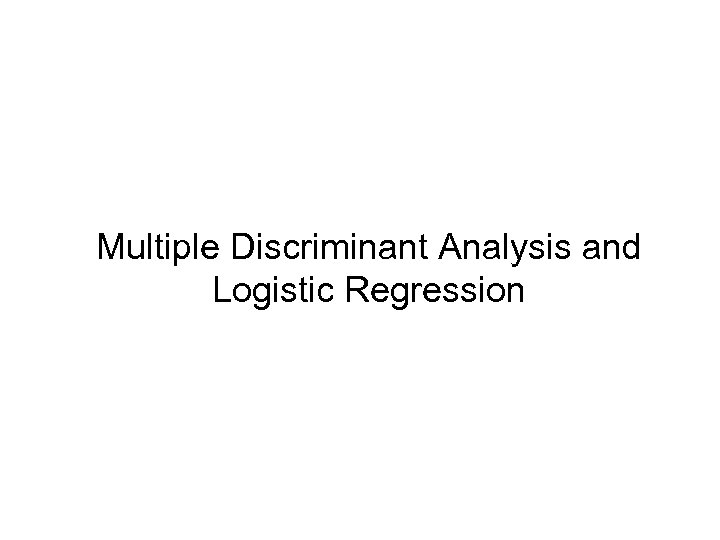Multiple Discriminant Analysis and Logistic RegressionMultiple Discriminant Analysis • Appropriate when dep. var. is categorical and indep. var. are metric • MDA derives variate that best distinguishes between a priori groups • MDA sets variate’s weights to maximize between-group variance relative to withingroup variance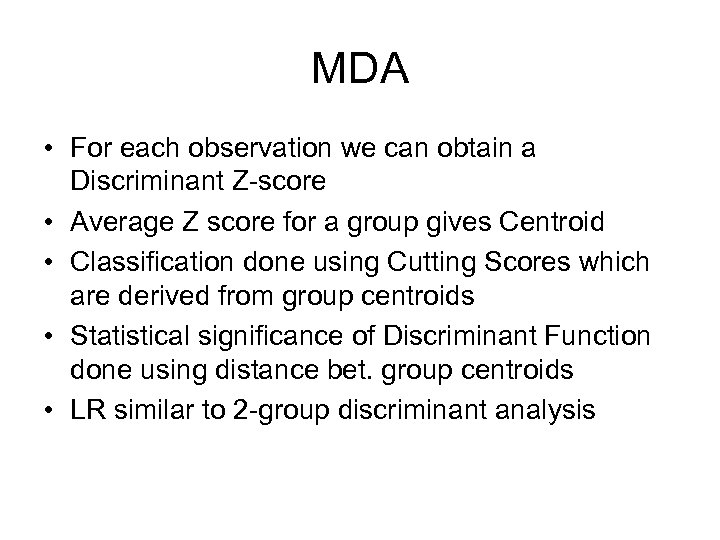MDA • For each observation we can obtain a Discriminant Z-score • Average Z score for a group gives Centroid • Classification done using Cutting Scores which are derived from group centroids • Statistical significance of Discriminant Function done using distance bet. group centroids • LR similar to 2 -group discriminant analysis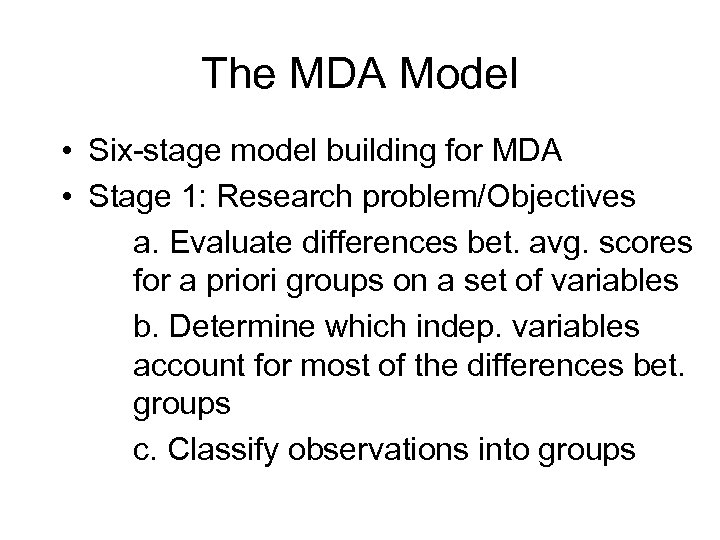The MDA Model • Six-stage model building for MDA • Stage 1: Research problem/Objectives a. Evaluate differences bet. avg. scores for a priori groups on a set of variables b. Determine which indep. variables account for most of the differences bet. groups c. Classify observations into groups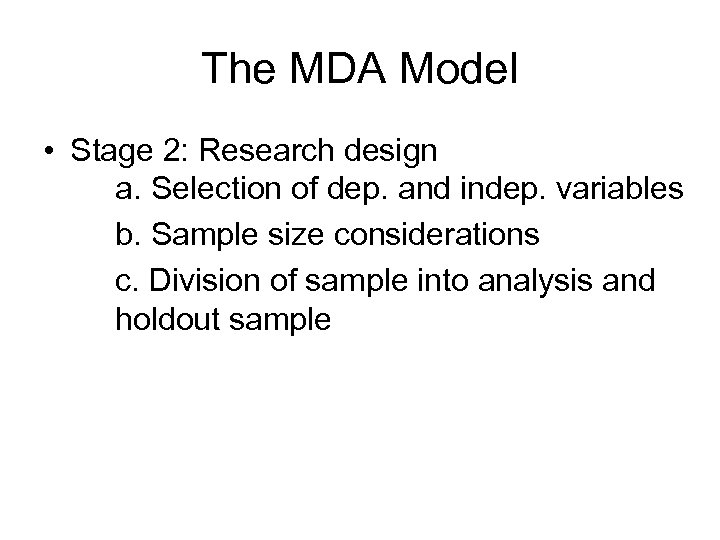The MDA Model • Stage 2: Research design a. Selection of dep. and indep. variables b. Sample size considerations c. Division of sample into analysis and holdout sample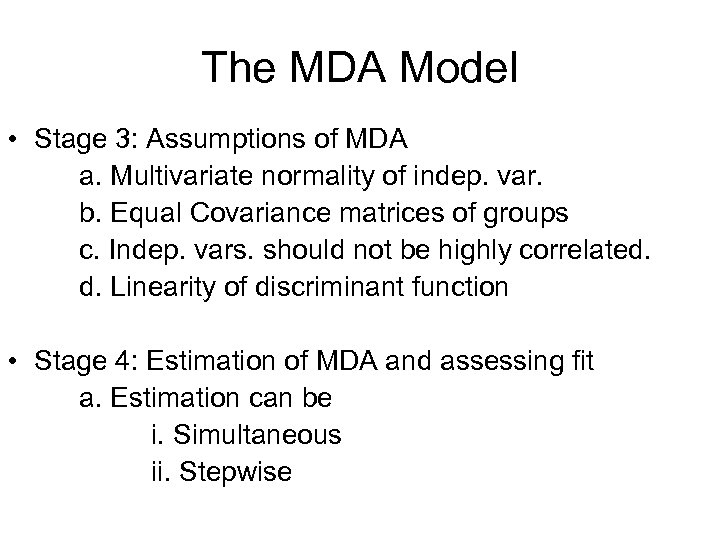The MDA Model • Stage 3: Assumptions of MDA a. Multivariate normality of indep. var. b. Equal Covariance matrices of groups c. Indep. vars. should not be highly correlated. d. Linearity of discriminant function • Stage 4: Estimation of MDA and assessing fit a. Estimation can be i. Simultaneous ii. Stepwise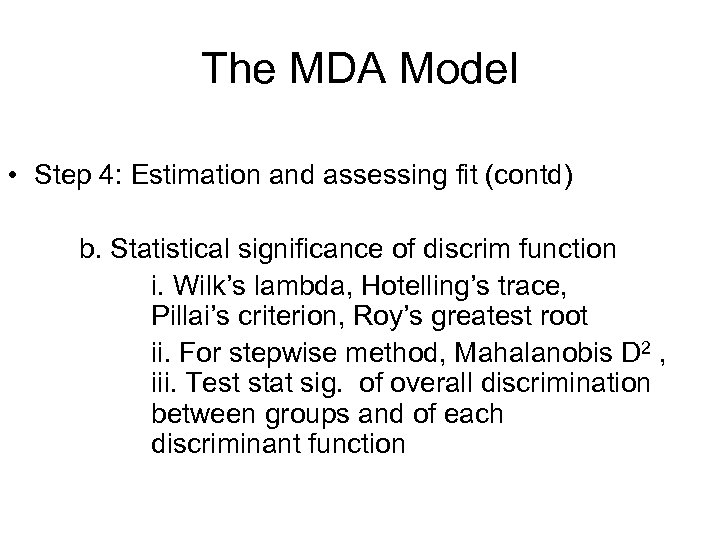The MDA Model • Step 4: Estimation and assessing fit (contd) b. Statistical significance of discrim function i. Wilk’s lambda, Hotelling’s trace, Pillai’s criterion, Roy’s greatest root ii. For stepwise method, Mahalanobis D 2 , iii. Test stat sig. of overall discrimination between groups and of each discriminant function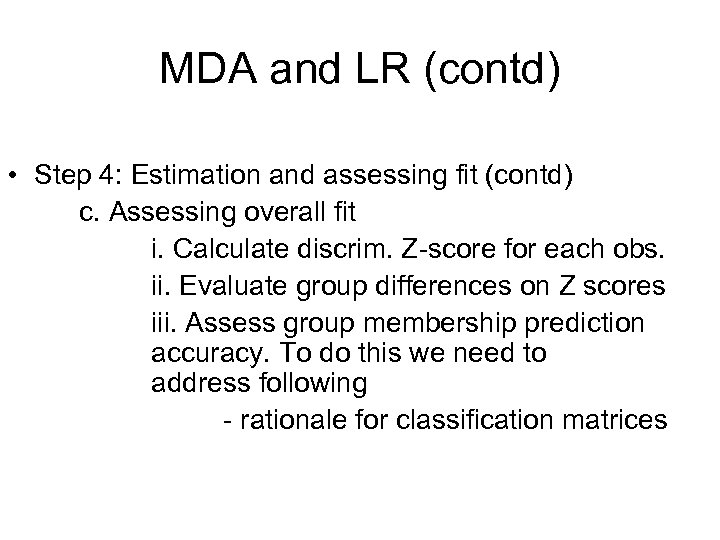MDA and LR (contd) • Step 4: Estimation and assessing fit (contd) c. Assessing overall fit i. Calculate discrim. Z-score for each obs. ii. Evaluate group differences on Z scores iii. Assess group membership prediction accuracy. To do this we need to address following - rationale for classification matrices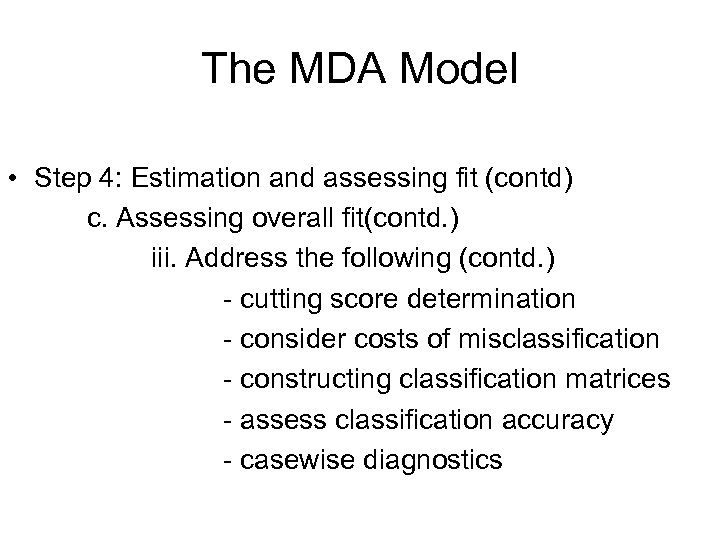The MDA Model • Step 4: Estimation and assessing fit (contd) c. Assessing overall fit(contd. ) iii. Address the following (contd. ) - cutting score determination - consider costs of misclassification - constructing classification matrices - assess classification accuracy - casewise diagnostics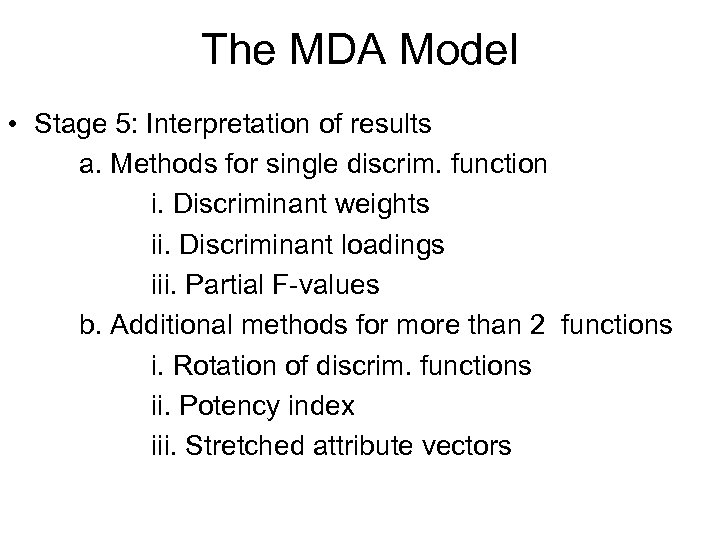The MDA Model • Stage 5: Interpretation of results a. Methods for single discrim. function i. Discriminant weights ii. Discriminant loadings iii. Partial F-values b. Additional methods for more than 2 functions i. Rotation of discrim. functions ii. Potency index iii. Stretched attribute vectors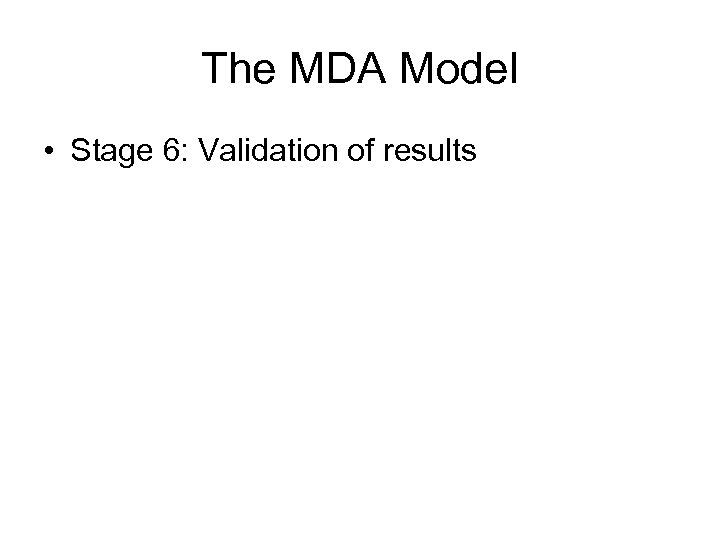The MDA Model • Stage 6: Validation of results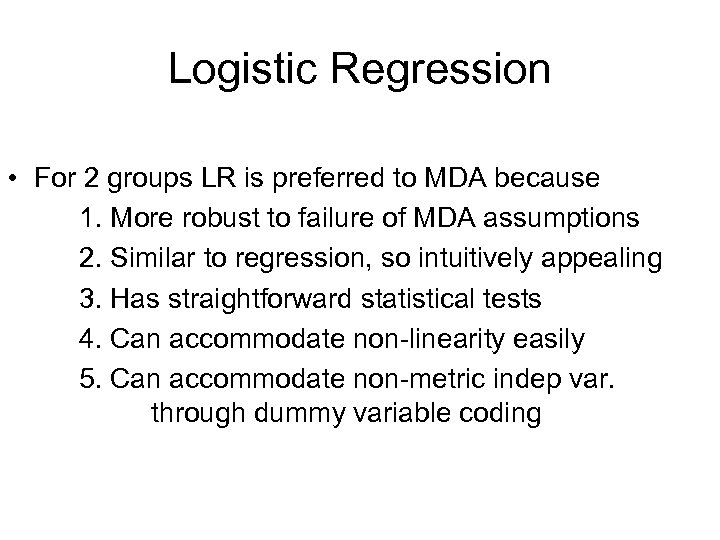Logistic Regression • For 2 groups LR is preferred to MDA because 1. More robust to failure of MDA assumptions 2. Similar to regression, so intuitively appealing 3. Has straightforward statistical tests 4. Can accommodate non-linearity easily 5. Can accommodate non-metric indep var. through dummy variable coding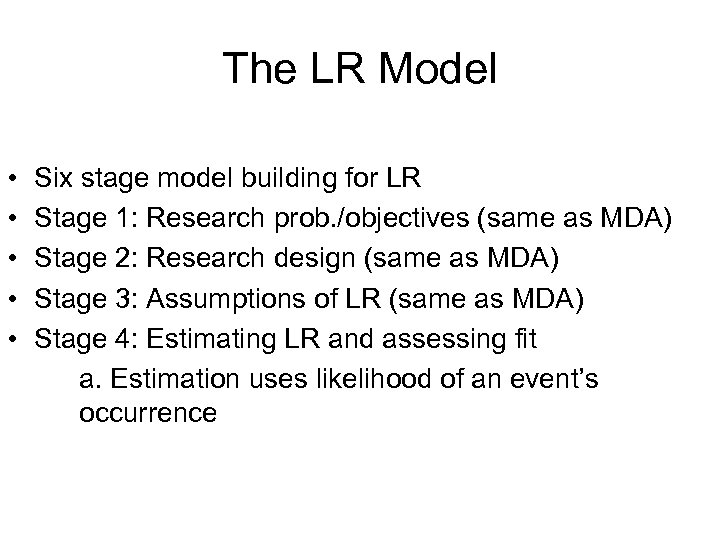The LR Model • • • Six stage model building for LR Stage 1: Research prob. /objectives (same as MDA) Stage 2: Research design (same as MDA) Stage 3: Assumptions of LR (same as MDA) Stage 4: Estimating LR and assessing fit a. Estimation uses likelihood of an event’s occurrence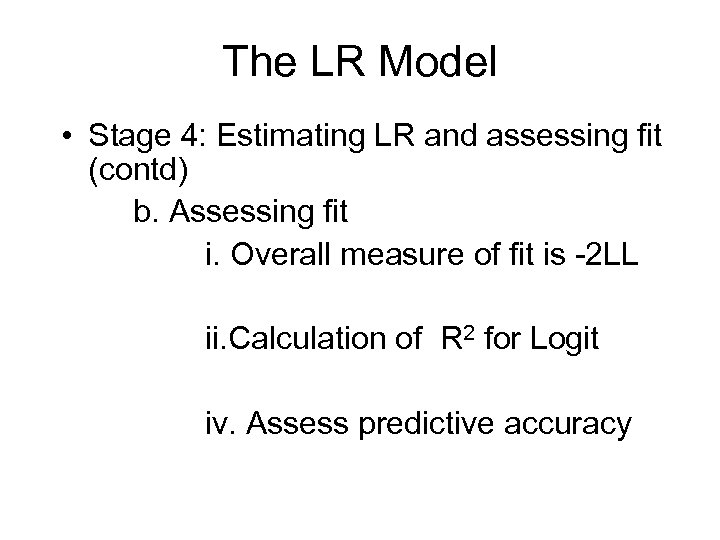The LR Model • Stage 4: Estimating LR and assessing fit (contd) b. Assessing fit i. Overall measure of fit is -2 LL ii. Calculation of R 2 for Logit iv. Assess predictive accuracy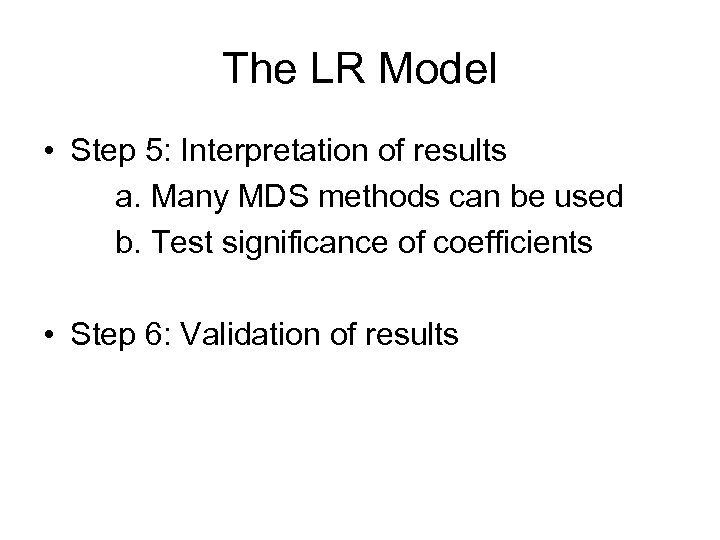The LR Model • Step 5: Interpretation of results a. Many MDS methods can be used b. Test significance of coefficients • Step 6: Validation of results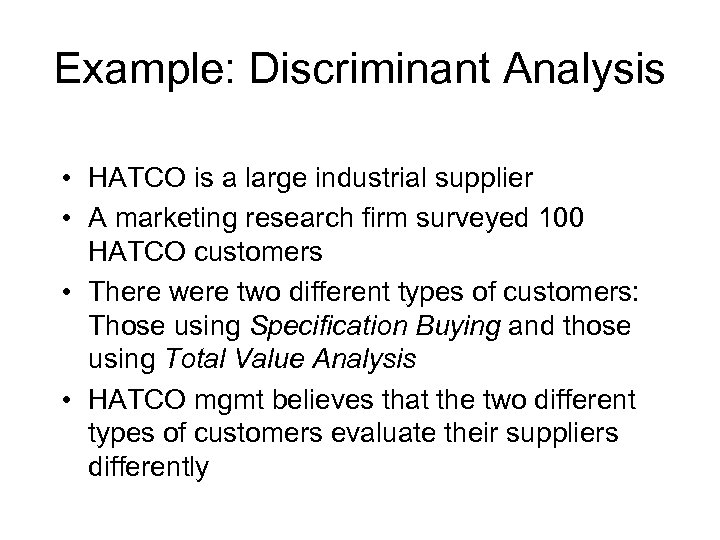Example: Discriminant Analysis • HATCO is a large industrial supplier • A marketing research firm surveyed 100 HATCO customers • There were two different types of customers: Those using Specification Buying and those using Total Value Analysis • HATCO mgmt believes that the two different types of customers evaluate their suppliers differently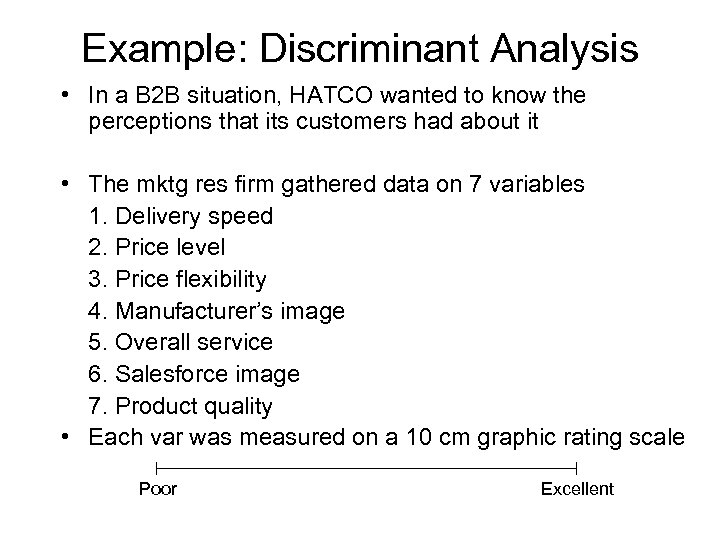Example: Discriminant Analysis • In a B 2 B situation, HATCO wanted to know the perceptions that its customers had about it • The mktg res firm gathered data on 7 variables 1. Delivery speed 2. Price level 3. Price flexibility 4. Manufacturer’s image 5. Overall service 6. Salesforce image 7. Product quality • Each var was measured on a 10 cm graphic rating scale Poor Excellent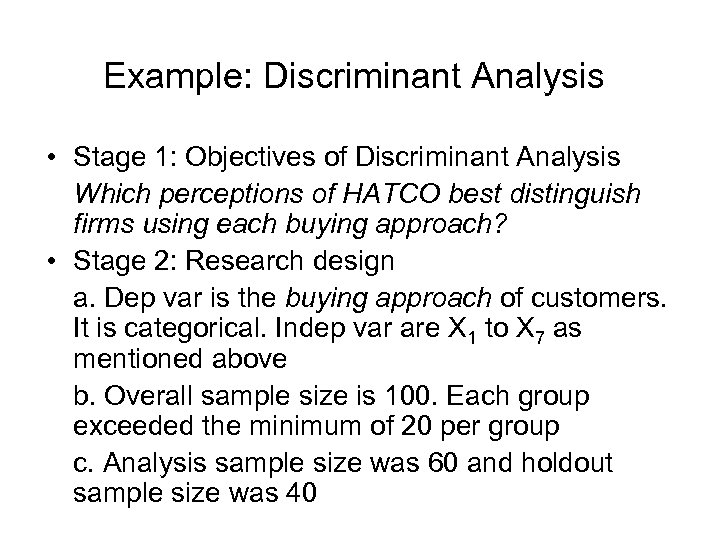Example: Discriminant Analysis • Stage 1: Objectives of Discriminant Analysis Which perceptions of HATCO best distinguish firms using each buying approach? • Stage 2: Research design a. Dep var is the buying approach of customers. It is categorical. Indep var are X 1 to X 7 as mentioned above b. Overall sample size is 100. Each group exceeded the minimum of 20 per group c. Analysis sample size was 60 and holdout sample size was 40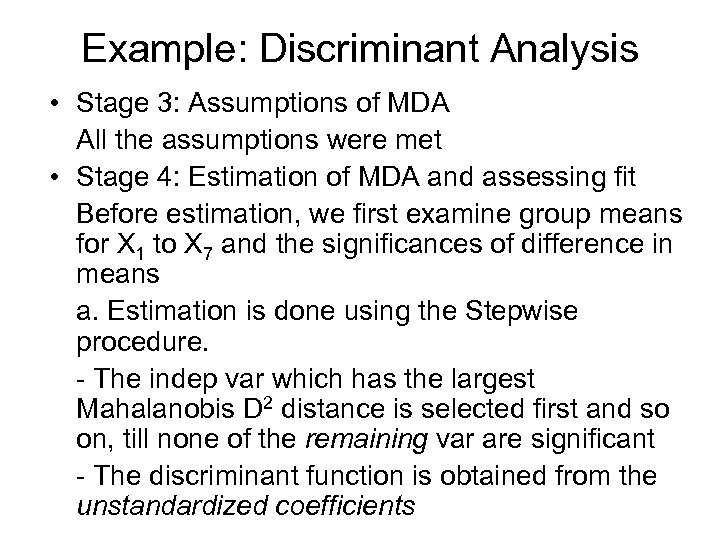Example: Discriminant Analysis • Stage 3: Assumptions of MDA All the assumptions were met • Stage 4: Estimation of MDA and assessing fit Before estimation, we first examine group means for X 1 to X 7 and the significances of difference in means a. Estimation is done using the Stepwise procedure. - The indep var which has the largest Mahalanobis D 2 distance is selected first and so on, till none of the remaining var are significant - The discriminant function is obtained from the unstandardized coefficients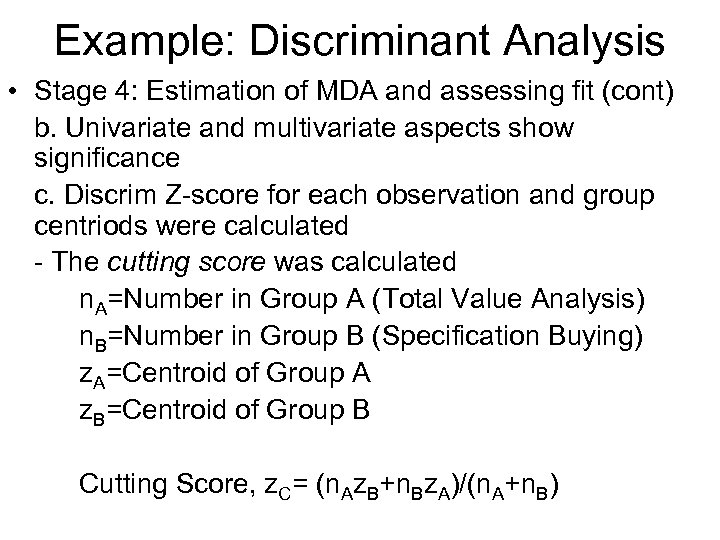Example: Discriminant Analysis • Stage 4: Estimation of MDA and assessing fit (cont) b. Univariate and multivariate aspects show significance c. Discrim Z-score for each observation and group centriods were calculated - The cutting score was calculated n. A=Number in Group A (Total Value Analysis) n. B=Number in Group B (Specification Buying) z. A=Centroid of Group A z. B=Centroid of Group B Cutting Score, z. C= (n. Az. B+n. Bz. A)/(n. A+n. B)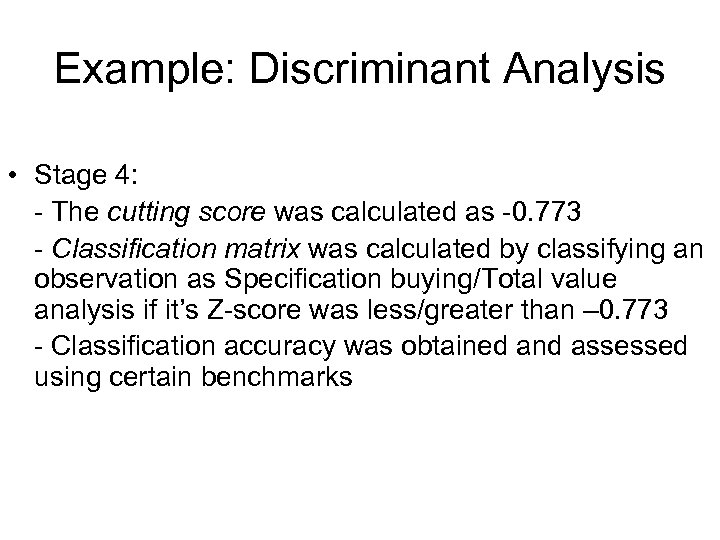Example: Discriminant Analysis • Stage 4: - The cutting score was calculated as -0. 773 - Classification matrix was calculated by classifying an observation as Specification buying/Total value analysis if it’s Z-score was less/greater than – 0. 773 - Classification accuracy was obtained and assessed using certain benchmarks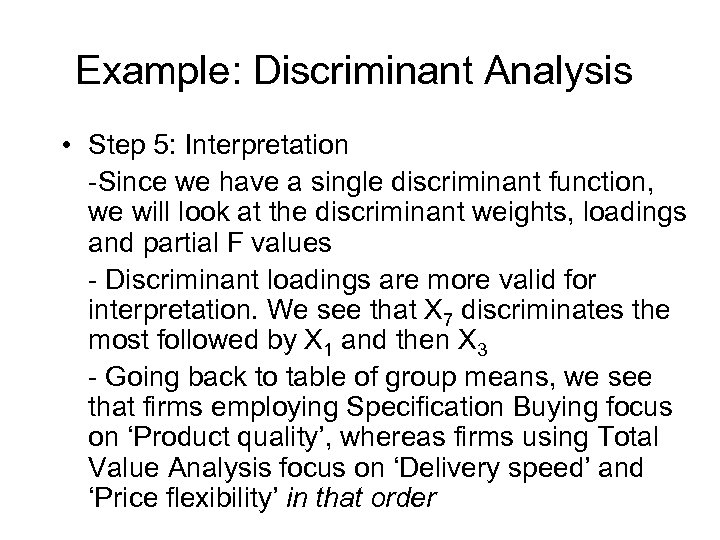Example: Discriminant Analysis • Step 5: Interpretation -Since we have a single discriminant function, we will look at the discriminant weights, loadings and partial F values - Discriminant loadings are more valid for interpretation. We see that X 7 discriminates the most followed by X 1 and then X 3 - Going back to table of group means, we see that firms employing Specification Buying focus on ‘Product quality’, whereas firms using Total Value Analysis focus on ‘Delivery speed’ and ‘Price flexibility’ in that order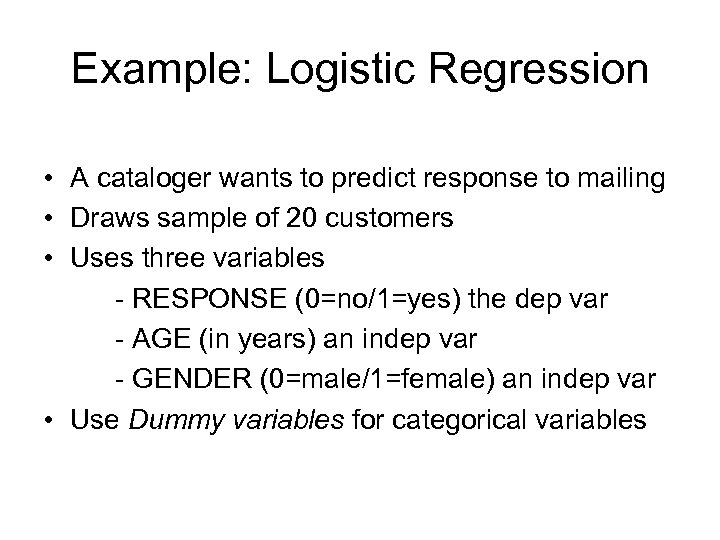Example: Logistic Regression • A cataloger wants to predict response to mailing • Draws sample of 20 customers • Uses three variables - RESPONSE (0=no/1=yes) the dep var - AGE (in years) an indep var - GENDER (0=male/1=female) an indep var • Use Dummy variables for categorical variables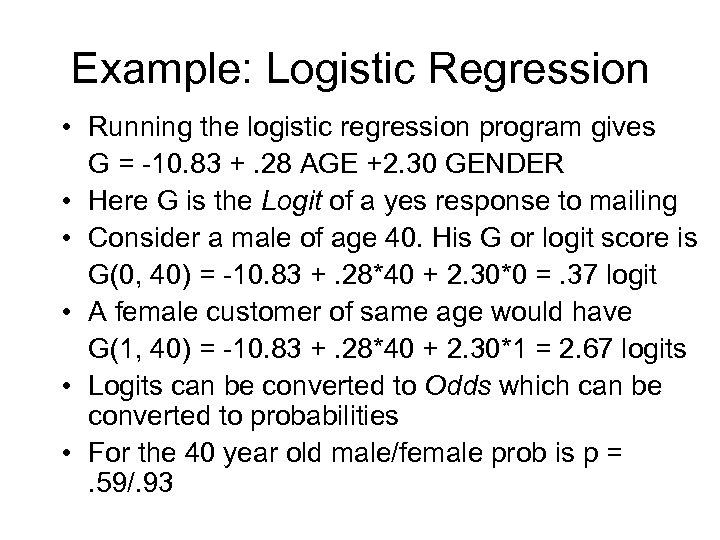Example: Logistic Regression • Running the logistic regression program gives G = -10. 83 +. 28 AGE +2. 30 GENDER • Here G is the Logit of a yes response to mailing • Consider a male of age 40. His G or logit score is G(0, 40) = -10. 83 +. 28*40 + 2. 30*0 =. 37 logit • A female customer of same age would have G(1, 40) = -10. 83 +. 28*40 + 2. 30*1 = 2. 67 logits • Logits can be converted to Odds which can be converted to probabilities • For the 40 year old male/female prob is p = . 59/. 93Factor Analysis © 2007 Prentice Hall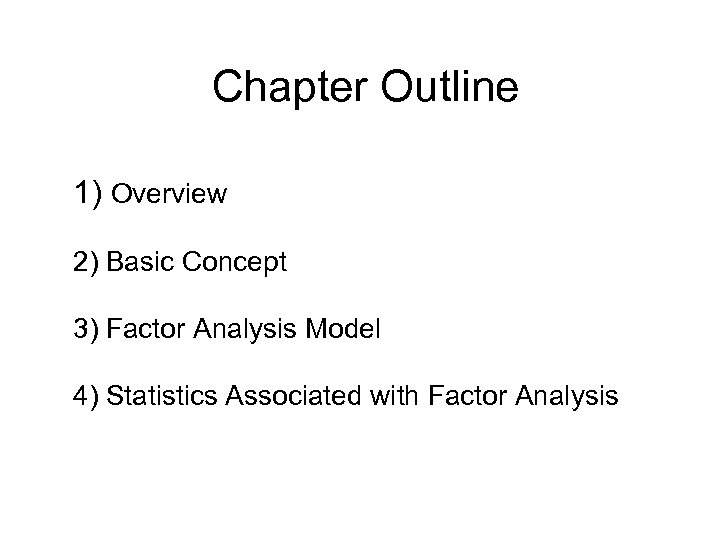Chapter Outline 1) Overview 2) Basic Concept 3) Factor Analysis Model 4) Statistics Associated with Factor Analysis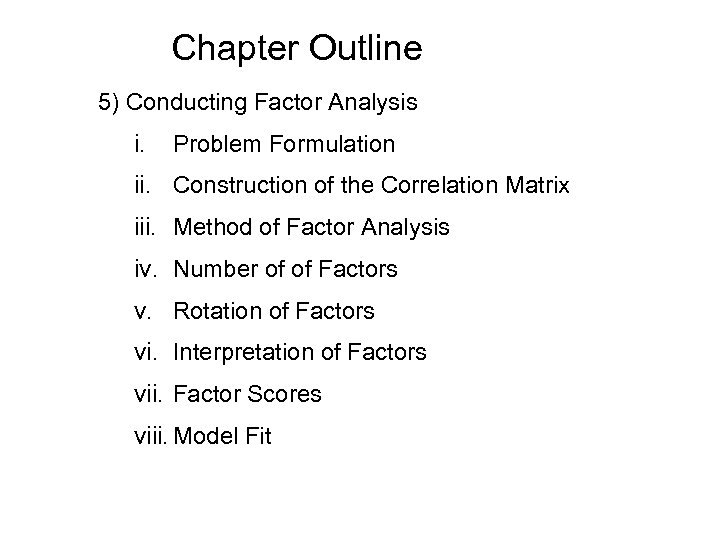Chapter Outline 5) Conducting Factor Analysis i. Problem Formulation ii. Construction of the Correlation Matrix iii. Method of Factor Analysis iv. Number of of Factors v. Rotation of Factors vi. Interpretation of Factors vii. Factor Scores viii. Model Fit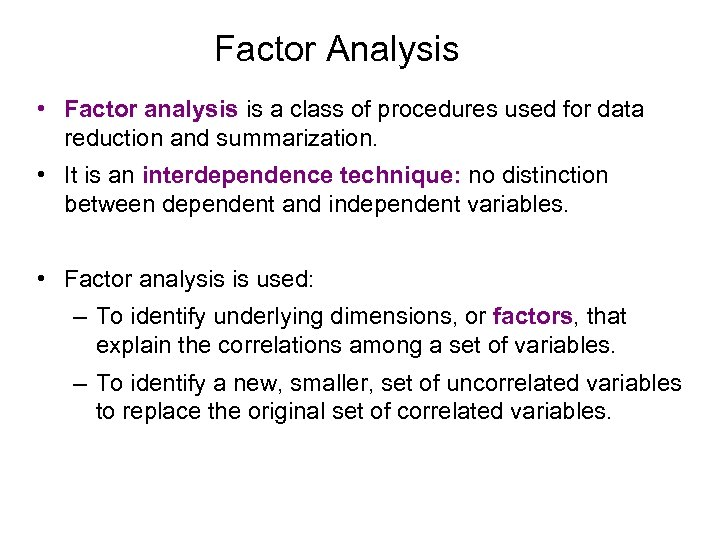Factor Analysis • Factor analysis is a class of procedures used for data reduction and summarization. • It is an interdependence technique: no distinction between dependent and independent variables. • Factor analysis is used: – To identify underlying dimensions, or factors, that explain the correlations among a set of variables. – To identify a new, smaller, set of uncorrelated variables to replace the original set of correlated variables.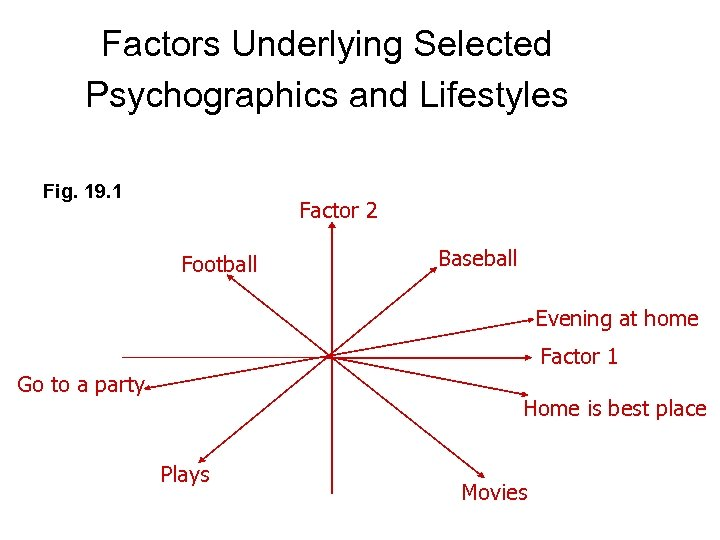Factors Underlying Selected Psychographics and Lifestyles Fig. 19. 1 Factor 2 Football Baseball Evening at home Factor 1 Go to a party Home is best place Plays Movies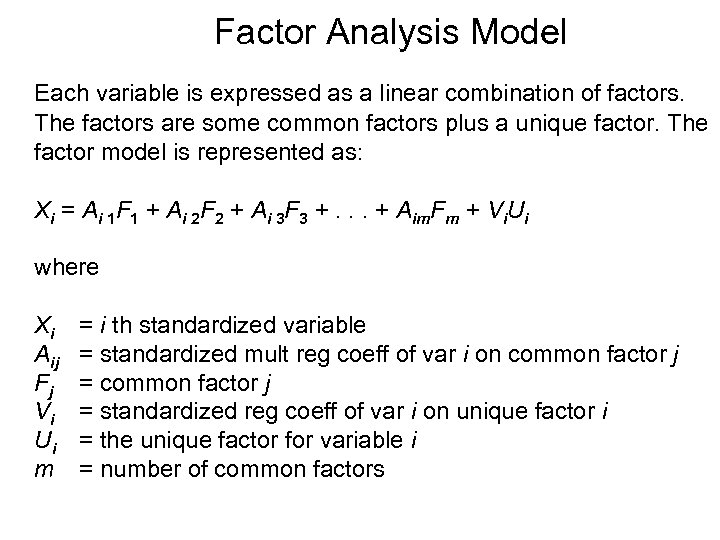Factor Analysis Model Each variable is expressed as a linear combination of factors. The factors are some common factors plus a unique factor. The factor model is represented as: Xi = Ai 1 F 1 + Ai 2 F 2 + Ai 3 F 3 +. . . + Aim. Fm + Vi. Ui where X i Aij Fj Vi Ui m = i th standardized variable = standardized mult reg coeff of var i on common factor j = standardized reg coeff of var i on unique factor i = the unique factor for variable i = number of common factors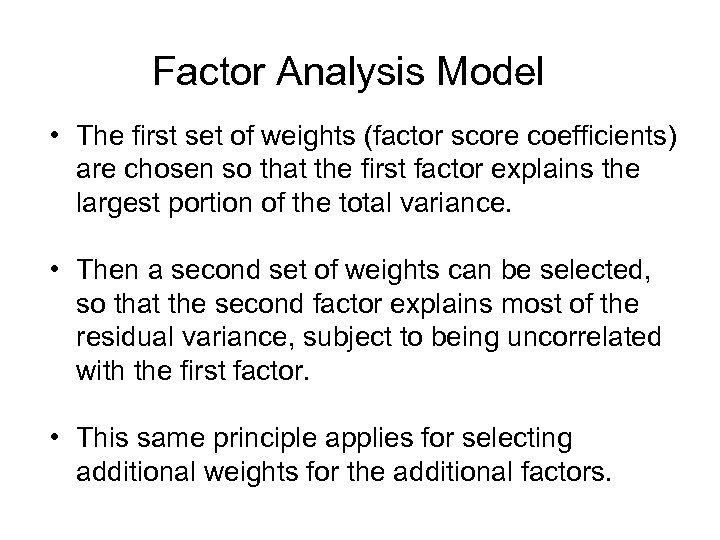Factor Analysis Model • The first set of weights (factor score coefficients) are chosen so that the first factor explains the largest portion of the total variance. • Then a second set of weights can be selected, so that the second factor explains most of the residual variance, subject to being uncorrelated with the first factor. • This same principle applies for selecting additional weights for the additional factors.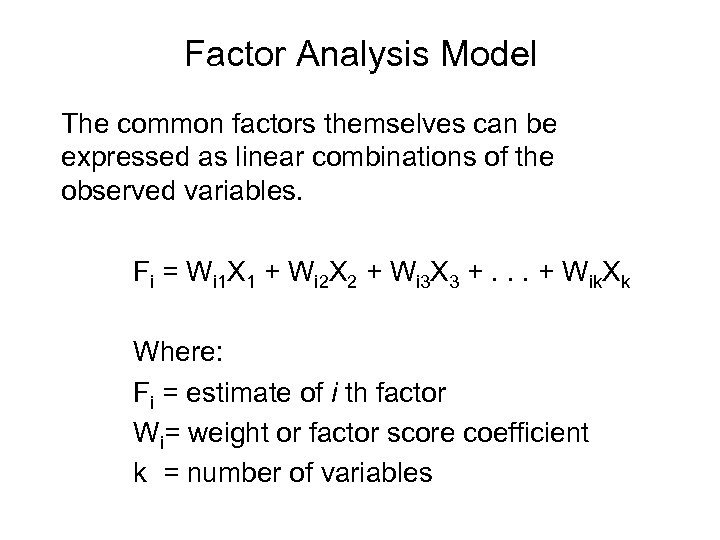Factor Analysis Model The common factors themselves can be expressed as linear combinations of the observed variables. Fi = Wi 1 X 1 + Wi 2 X 2 + Wi 3 X 3 +. . . + Wik. Xk Where: Fi = estimate of i th factor Wi= weight or factor score coefficient k = number of variables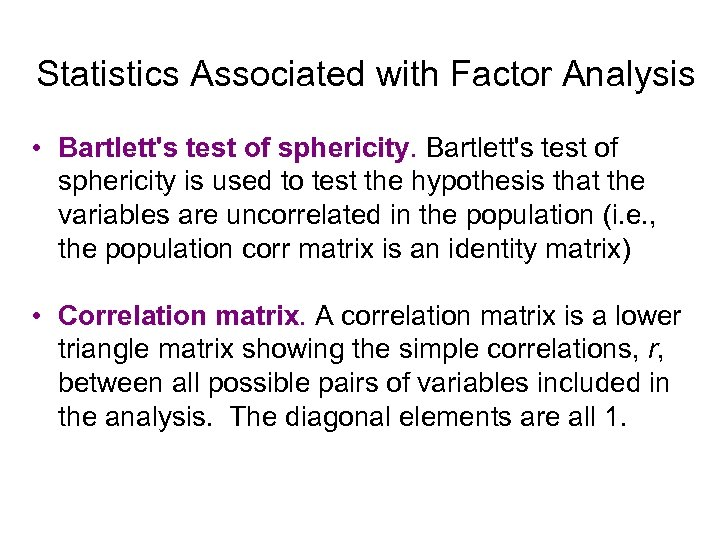Statistics Associated with Factor Analysis • Bartlett's test of sphericity is used to test the hypothesis that the variables are uncorrelated in the population (i. e. , the population corr matrix is an identity matrix) • Correlation matrix. A correlation matrix is a lower triangle matrix showing the simple correlations, r, between all possible pairs of variables included in the analysis. The diagonal elements are all 1.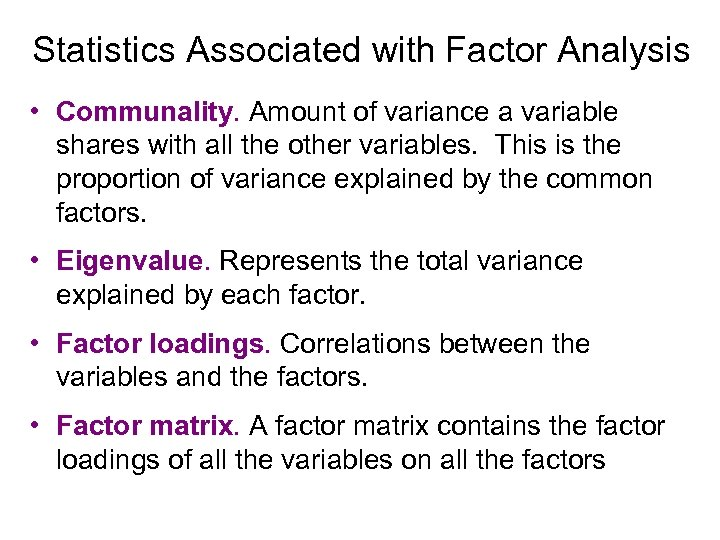Statistics Associated with Factor Analysis • Communality. Amount of variance a variable shares with all the other variables. This is the proportion of variance explained by the common factors. • Eigenvalue. Represents the total variance explained by each factor. • Factor loadings. Correlations between the variables and the factors. • Factor matrix. A factor matrix contains the factor loadings of all the variables on all the factors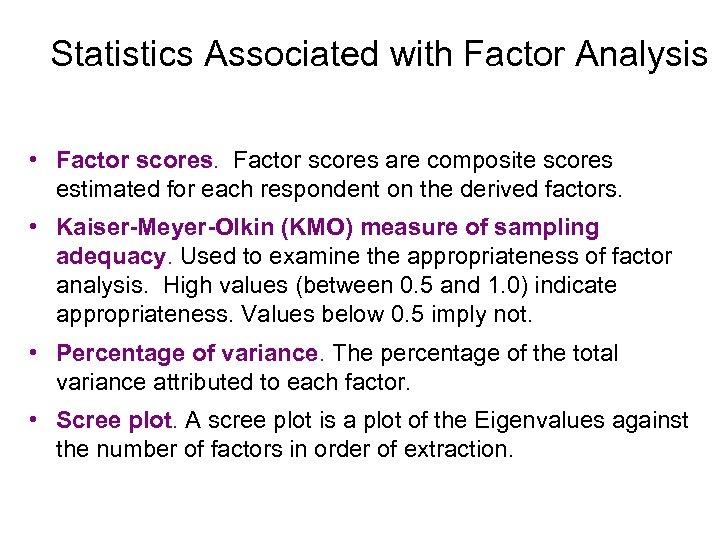Statistics Associated with Factor Analysis • Factor scores are composite scores estimated for each respondent on the derived factors. • Kaiser-Meyer-Olkin (KMO) measure of sampling adequacy. Used to examine the appropriateness of factor analysis. High values (between 0. 5 and 1. 0) indicate appropriateness. Values below 0. 5 imply not. • Percentage of variance. The percentage of the total variance attributed to each factor. • Scree plot. A scree plot is a plot of the Eigenvalues against the number of factors in order of extraction.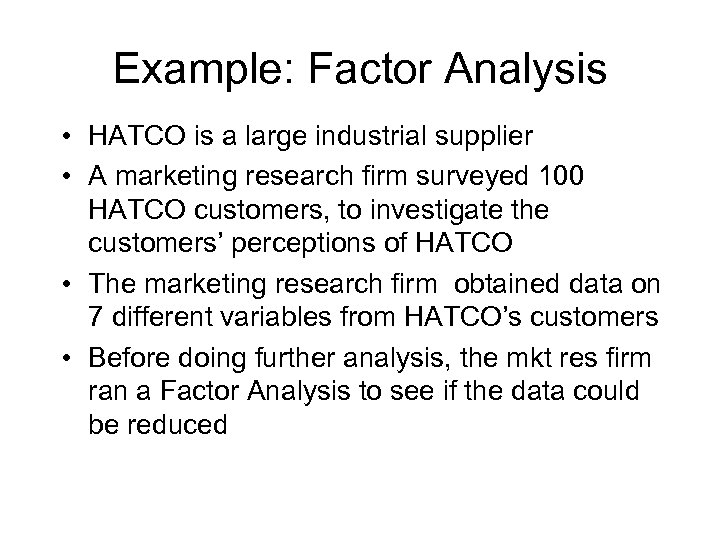Example: Factor Analysis • HATCO is a large industrial supplier • A marketing research firm surveyed 100 HATCO customers, to investigate the customers’ perceptions of HATCO • The marketing research firm obtained data on 7 different variables from HATCO’s customers • Before doing further analysis, the mkt res firm ran a Factor Analysis to see if the data could be reduced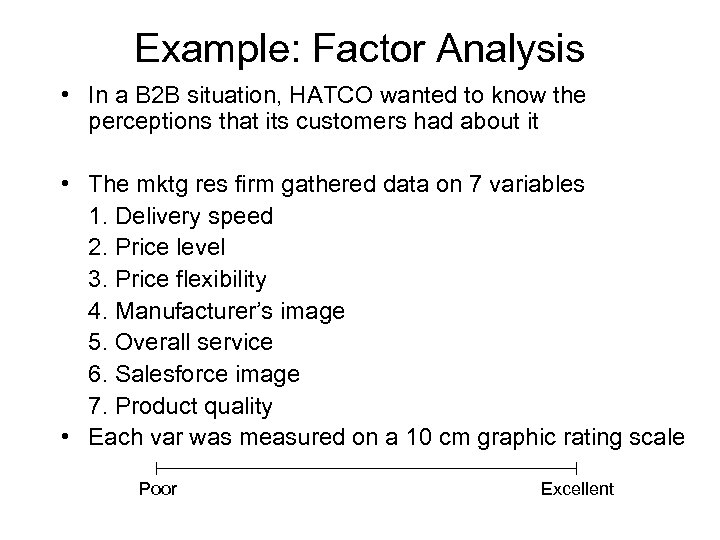Example: Factor Analysis • In a B 2 B situation, HATCO wanted to know the perceptions that its customers had about it • The mktg res firm gathered data on 7 variables 1. Delivery speed 2. Price level 3. Price flexibility 4. Manufacturer’s image 5. Overall service 6. Salesforce image 7. Product quality • Each var was measured on a 10 cm graphic rating scale Poor Excellent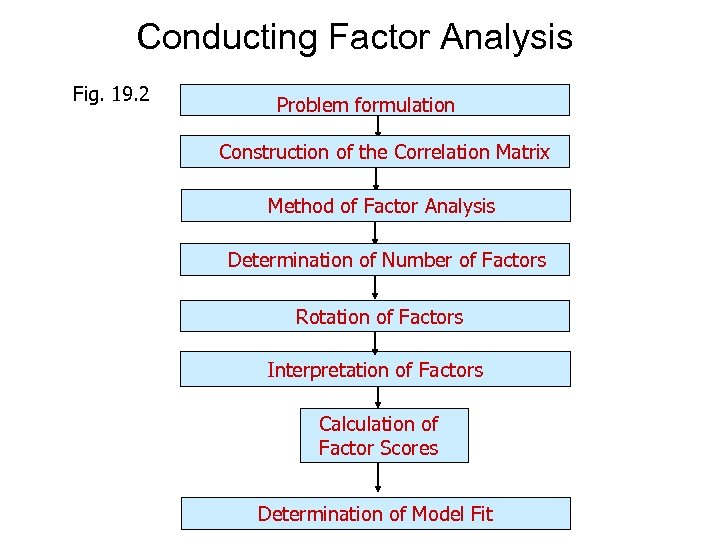Conducting Factor Analysis Fig. 19. 2 Problem formulation Construction of the Correlation Matrix Method of Factor Analysis Determination of Number of Factors Rotation of Factors Interpretation of Factors Calculation of Factor Scores Determination of Model Fit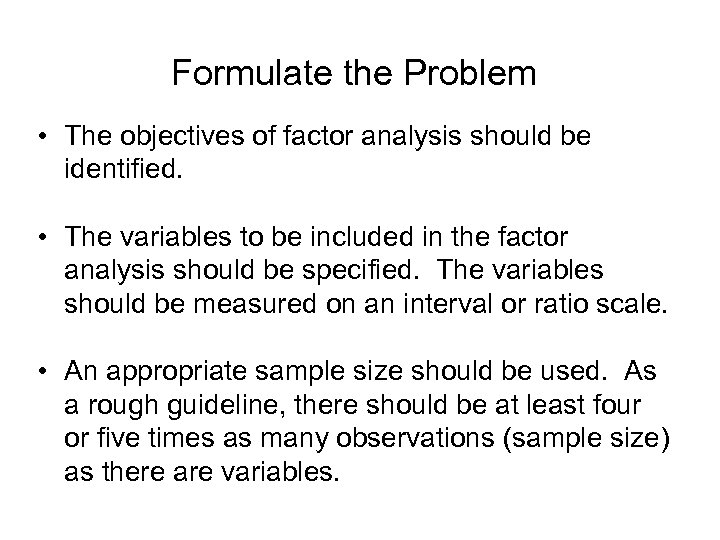Formulate the Problem • The objectives of factor analysis should be identified. • The variables to be included in the factor analysis should be specified. The variables should be measured on an interval or ratio scale. • An appropriate sample size should be used. As a rough guideline, there should be at least four or five times as many observations (sample size) as there are variables.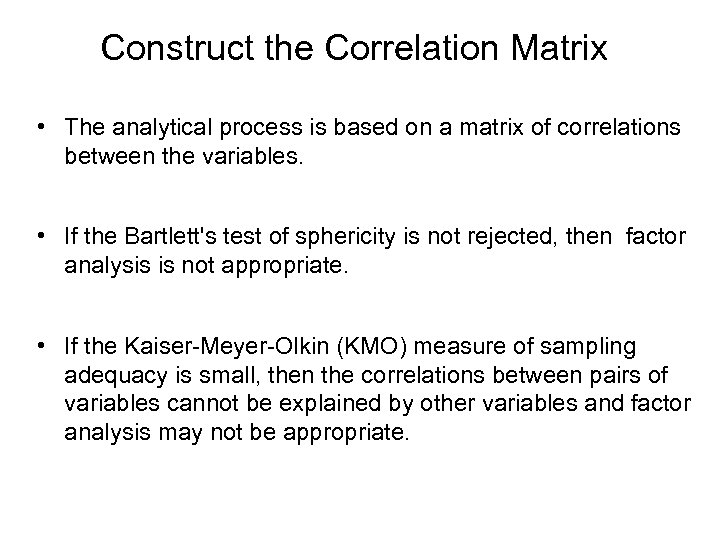Construct the Correlation Matrix • The analytical process is based on a matrix of correlations between the variables. • If the Bartlett's test of sphericity is not rejected, then factor analysis is not appropriate. • If the Kaiser-Meyer-Olkin (KMO) measure of sampling adequacy is small, then the correlations between pairs of variables cannot be explained by other variables and factor analysis may not be appropriate.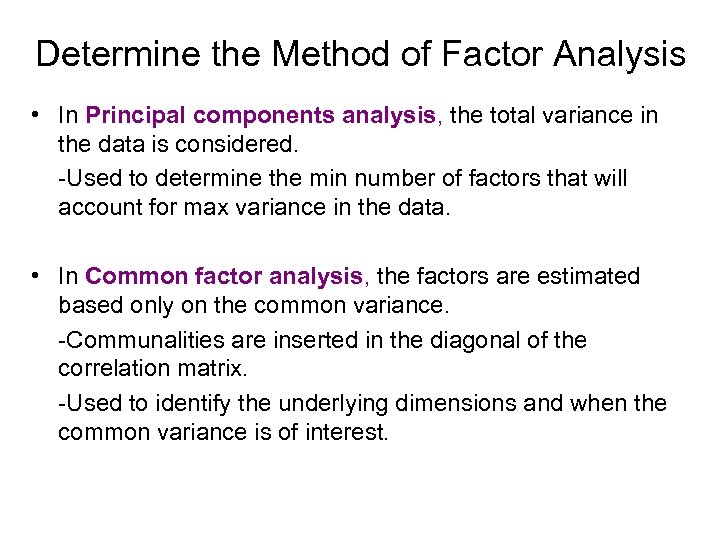Determine the Method of Factor Analysis • In Principal components analysis, the total variance in the data is considered. -Used to determine the min number of factors that will account for max variance in the data. • In Common factor analysis, the factors are estimated based only on the common variance. -Communalities are inserted in the diagonal of the correlation matrix. -Used to identify the underlying dimensions and when the common variance is of interest.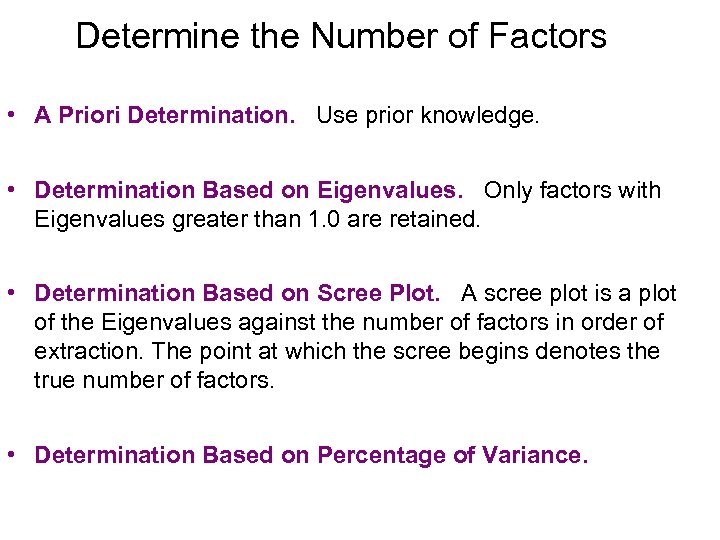Determine the Number of Factors • A Priori Determination. Use prior knowledge. • Determination Based on Eigenvalues. Only factors with Eigenvalues greater than 1. 0 are retained. • Determination Based on Scree Plot. A scree plot is a plot of the Eigenvalues against the number of factors in order of extraction. The point at which the scree begins denotes the true number of factors. • Determination Based on Percentage of Variance.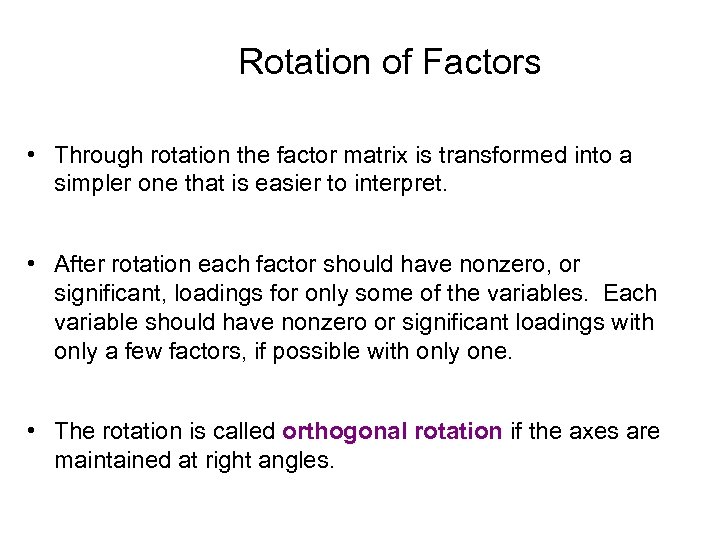Rotation of Factors • Through rotation the factor matrix is transformed into a simpler one that is easier to interpret. • After rotation each factor should have nonzero, or significant, loadings for only some of the variables. Each variable should have nonzero or significant loadings with only a few factors, if possible with only one. • The rotation is called orthogonal rotation if the axes are maintained at right angles.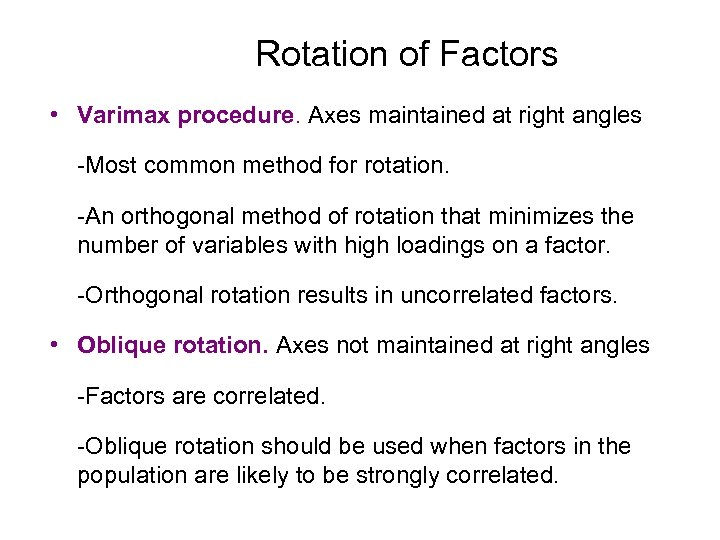Rotation of Factors • Varimax procedure. Axes maintained at right angles -Most common method for rotation. -An orthogonal method of rotation that minimizes the number of variables with high loadings on a factor. -Orthogonal rotation results in uncorrelated factors. • Oblique rotation. Axes not maintained at right angles -Factors are correlated. -Oblique rotation should be used when factors in the population are likely to be strongly correlated.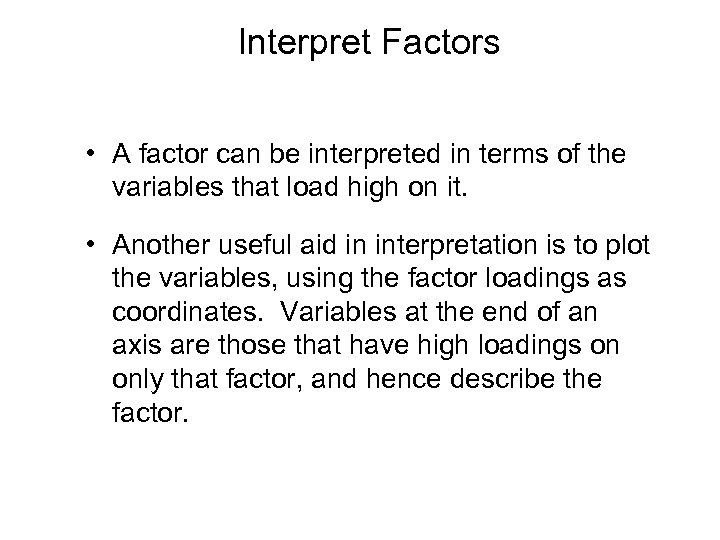Interpret Factors • A factor can be interpreted in terms of the variables that load high on it. • Another useful aid in interpretation is to plot the variables, using the factor loadings as coordinates. Variables at the end of an axis are those that have high loadings on only that factor, and hence describe the factor.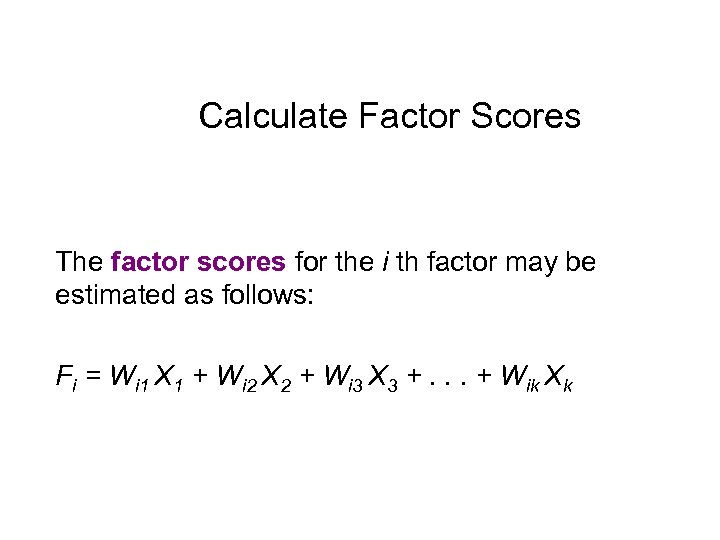Calculate Factor Scores The factor scores for the i th factor may be estimated as follows: Fi = Wi 1 X 1 + Wi 2 X 2 + Wi 3 X 3 +. . . + Wik Xk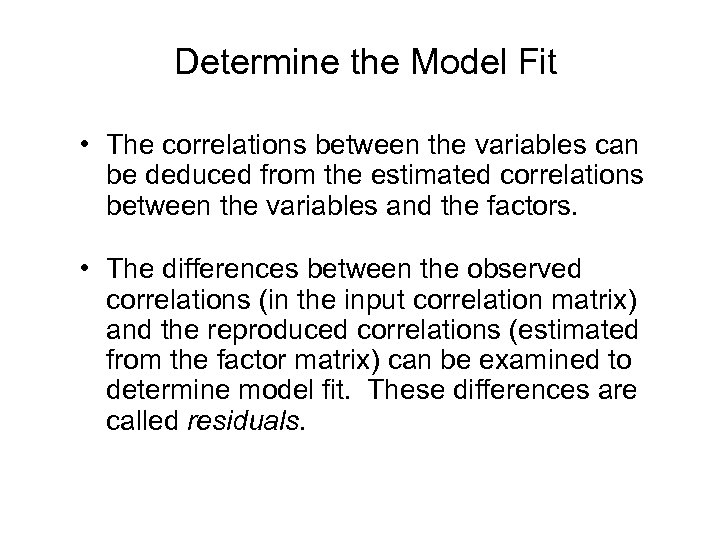Determine the Model Fit • The correlations between the variables can be deduced from the estimated correlations between the variables and the factors. • The differences between the observed correlations (in the input correlation matrix) and the reproduced correlations (estimated from the factor matrix) can be examined to determine model fit. These differences are called residuals.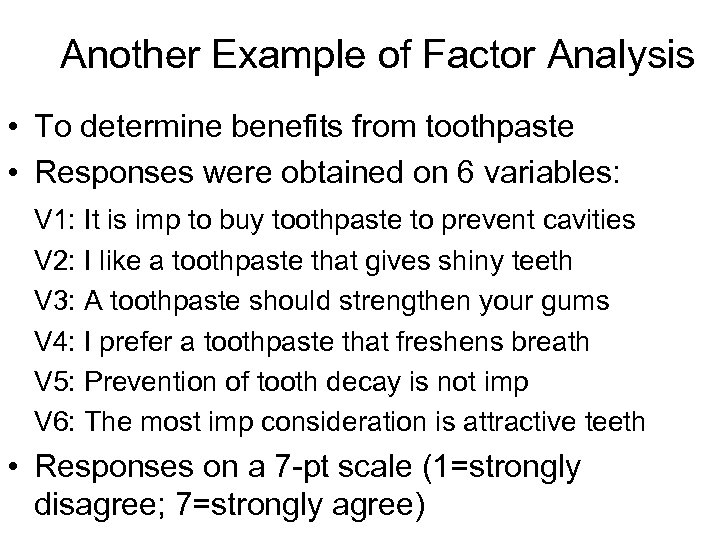Another Example of Factor Analysis • To determine benefits from toothpaste • Responses were obtained on 6 variables: V 1: It is imp to buy toothpaste to prevent cavities V 2: I like a toothpaste that gives shiny teeth V 3: A toothpaste should strengthen your gums V 4: I prefer a toothpaste that freshens breath V 5: Prevention of tooth decay is not imp V 6: The most imp consideration is attractive teeth • Responses on a 7 -pt scale (1=strongly disagree; 7=strongly agree)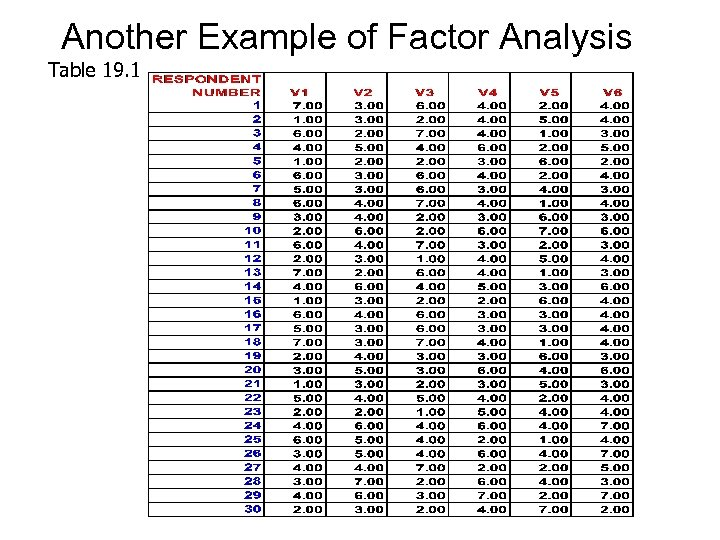Another Example of Factor Analysis Table 19. 1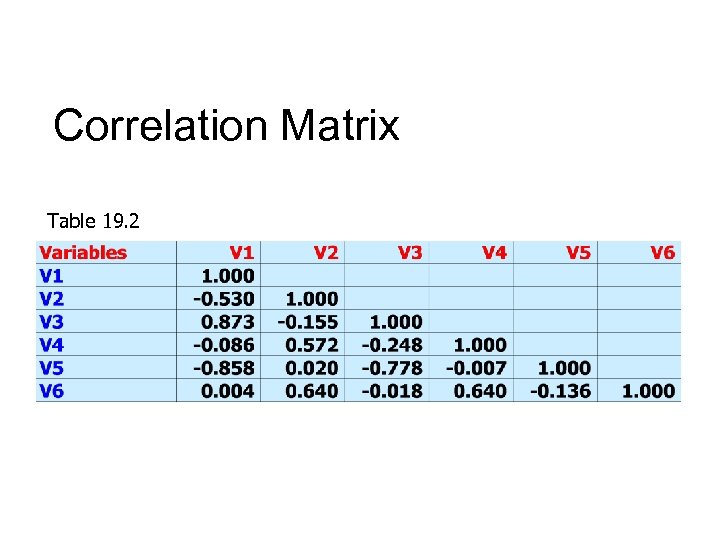Correlation Matrix Table 19. 2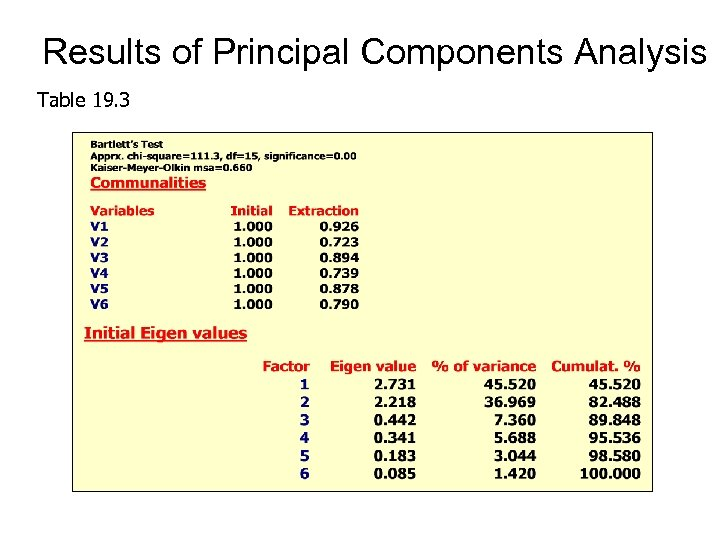Results of Principal Components Analysis Table 19. 3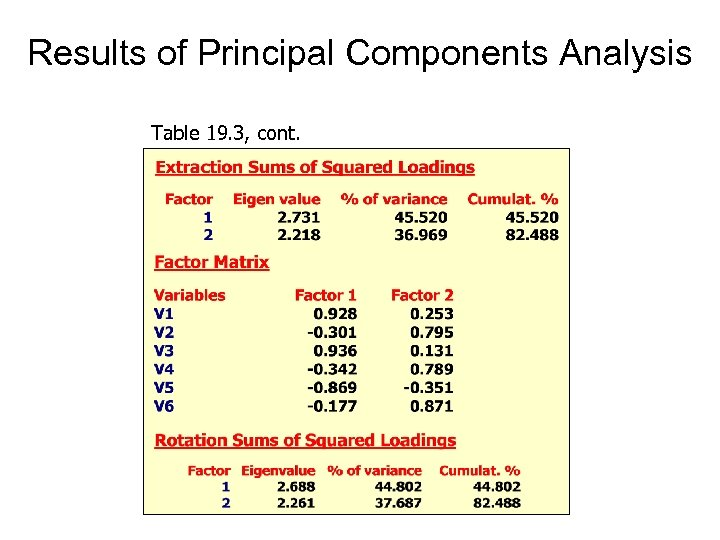Results of Principal Components Analysis Table 19. 3, cont.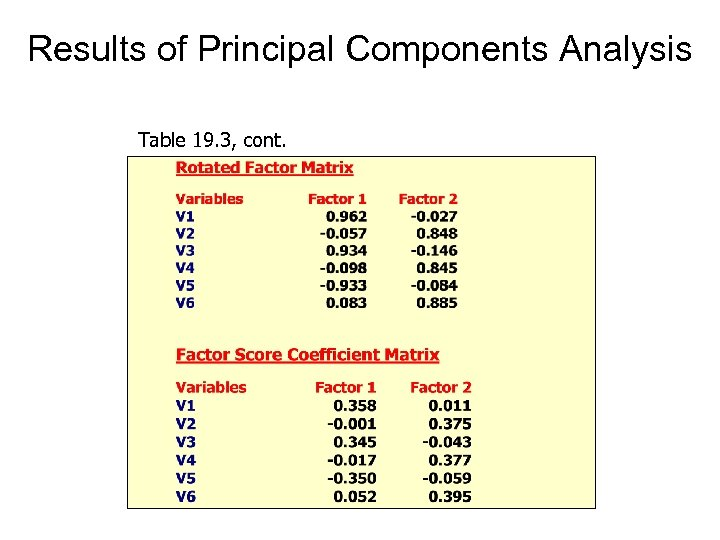Results of Principal Components Analysis Table 19. 3, cont.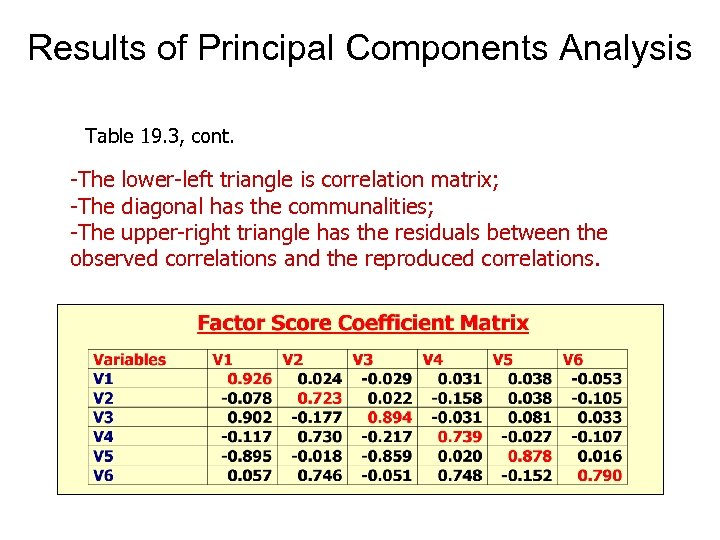Results of Principal Components Analysis Table 19. 3, cont. -The lower-left triangle is correlation matrix; -The diagonal has the communalities; -The upper-right triangle has the residuals between the observed correlations and the reproduced correlations.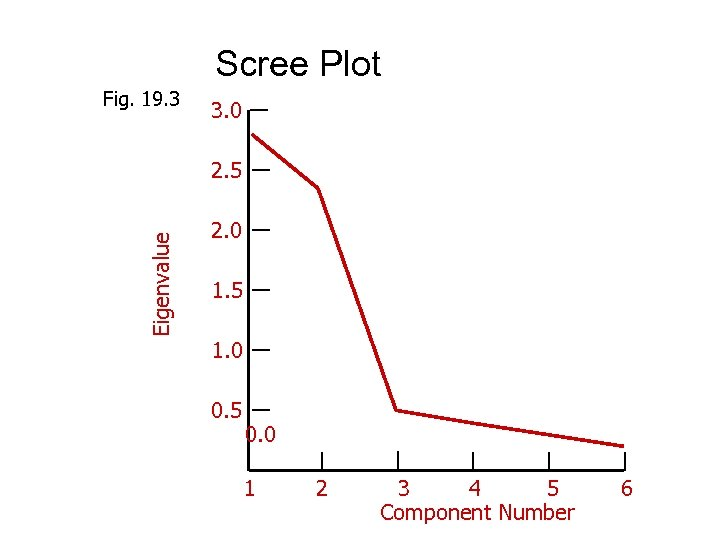Scree Plot Fig. 19. 3 3. 0 Eigenvalue 2. 5 2. 0 1. 5 1. 0 0. 5 0. 0 1 2 3 4 5 Component Number 6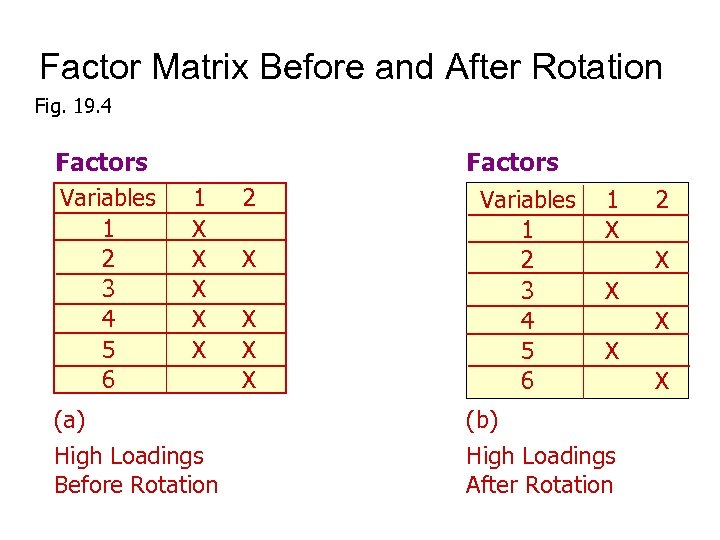Factor Matrix Before and After Rotation Fig. 19. 4 Factors Variables 1 2 3 4 5 6 1 X X X (a) High Loadings Before Rotation 2 X X Factors Variables 1 2 3 4 5 6 1 X 2 X X (b) High Loadings After Rotation X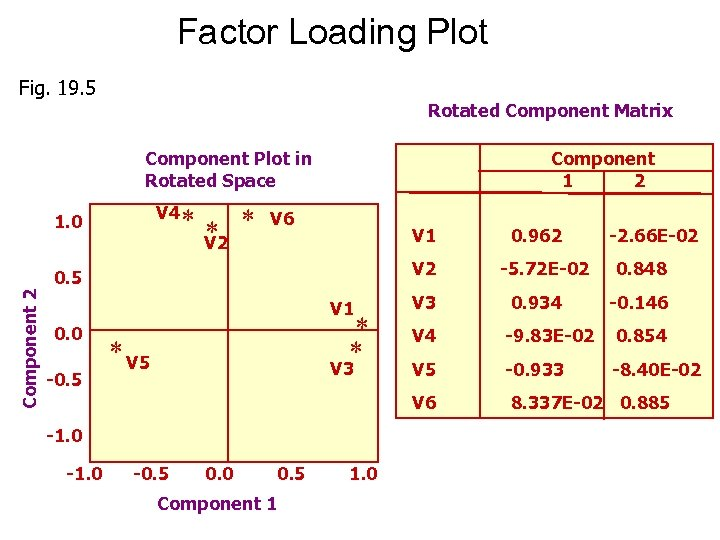Factor Loading Plot Fig. 19. 5 Rotated Component Matrix Component 1 2 Component Plot in Rotated Space V 4 1. 0 ** * V 2 V 6 V 1 V 2 Component 2 0. 5 0. 962 -5. 72 E-02 -2. 66 E-02 0. 848 0. 0 -0. 5 * * * V 5 V 3 -1. 0 -0. 5 0. 0 0. 5 Component 1 1. 0 V 3 0. 934 V 4 -9. 83 E-02 0. 854 V 5 -0. 933 -8. 40 E-02 V 6 V 1 -0. 146 8. 337 E-02 0. 885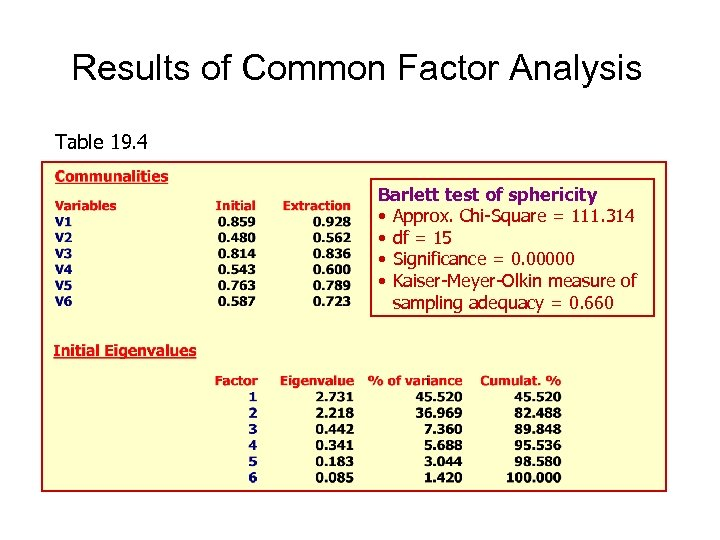Results of Common Factor Analysis Table 19. 4 Barlett test of sphericity • Approx. Chi-Square = 111. 314 • df = 15 • Significance = 0. 00000 • Kaiser-Meyer-Olkin measure of sampling adequacy = 0. 660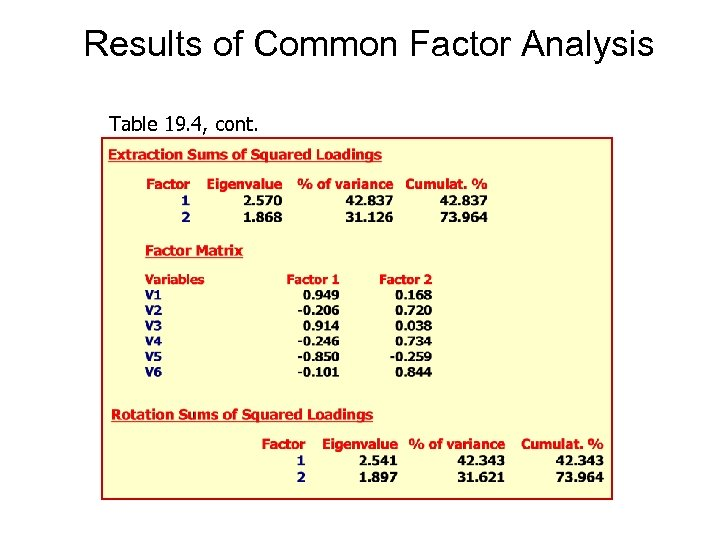Results of Common Factor Analysis Table 19. 4, cont.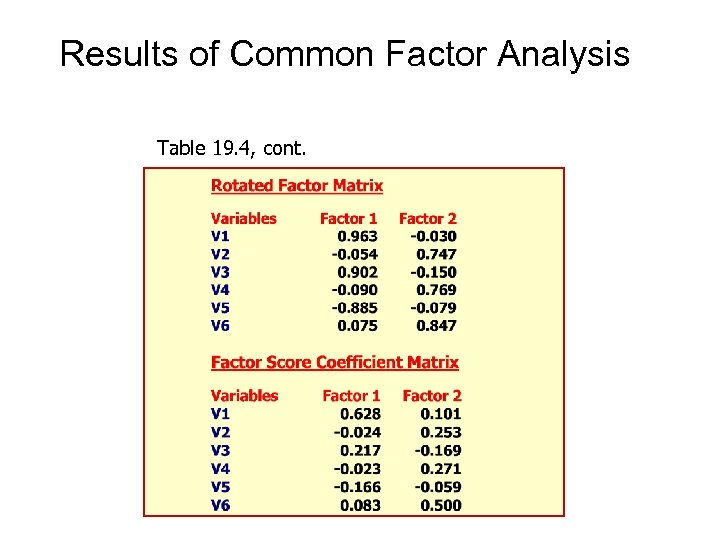Results of Common Factor Analysis Table 19. 4, cont.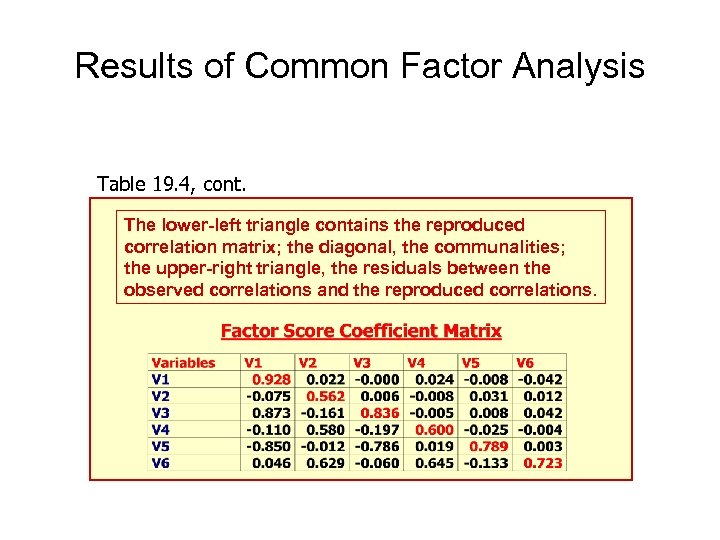Results of Common Factor Analysis Table 19. 4, cont. The lower-left triangle contains the reproduced correlation matrix; the diagonal, the communalities; the upper-right triangle, the residuals between the observed correlations and the reproduced correlations.